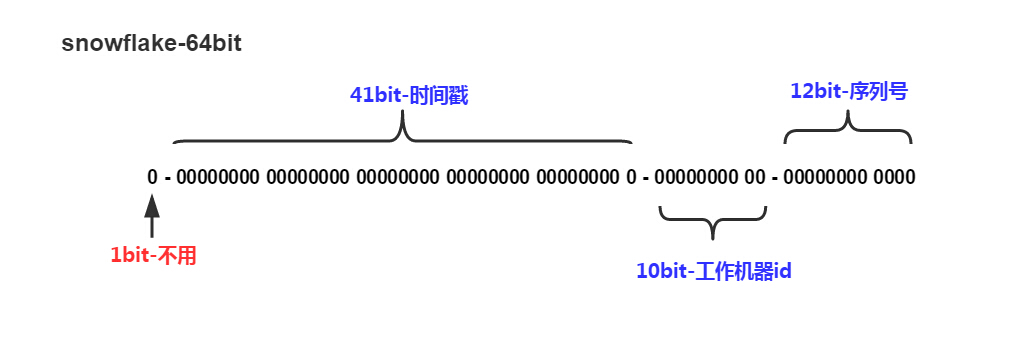# 短网址服务(TinyURL)生成算法

前不久做了一个优惠劵的分享功能，其中一个功能就是生成一个优惠劵分享短链接。生成的短链接要求每个链接都是唯一的，并且长度尽可能短。在网上查了一下相关的思路，发现了一个不错的算法。这个算法的思路就是用[a-zA-Z0-9]建立一个长度为62的矩阵，然后把矩阵打乱，再生成一个全局唯一的数字，再把这个数字用矩阵内的元素表示转换成62进制,生成的链接长度最大才11位。所以短链接的生成关键点就变成了如何生成一个全局唯一的数字和实现进制的转换。

## 1、生成全局唯一的数字

这本质是一个分布式ID的问题。如果简单处理的话可以借用redis的incr操作这样每次取到的ID都是单调递增且唯一的。另外一种方式是借用mysql,这里不是借用mysql的主键的auto_incr特性。而是每一台应用来请求时分配一个范围比如 s1 [100-200], s2 来请求的时候就分配 [201-301]，本质是利用乐观锁进行一个cas操作。

如果不想借助外部去生成ID的话，可以用UUID算法。UUID长度12个字节组成由，以下几个部分组成。

• 4个字节表示的Unix timestamp,
• 3个字节表示的机器的ID
• 2个字节表示的进程ID
• 3个字节表示的计数器

UUID是一类算法的统称，具体有不同的实现。优点是每台机器可以独立产生ID，理论上保证不会重复，所以天然是分布式的，缺点是生成的ID太长，不仅占用内存，而且索引查询效率低。## 2、进制转换

这个操作就相对简单了。直接上代码 ~

/**
* 短链接生成
*/
public class TinyURL {

public static final char[] array =
{'0', '1', '2', '3', '4', '5', '6', '7', '8', '9',
'q', 'w', 'e', 'r', 't', 'y', 'u', 'i', 'o', 'p', 'a', 's', 'd',
'f', 'g', 'h', 'j', 'k', 'l', 'z', 'x', 'c', 'v', 'b', 'n', 'm',
'Q', 'W', 'E', 'R', 'T', 'Y', 'U', 'I', 'O', 'P', 'A', 'S', 'D',
'F', 'G', 'H', 'J', 'K', 'L', 'Z', 'X', 'C', 'V', 'B', 'N', 'M'};

public static Map<Character, Integer> charValueMap = new HashMap<Character, Integer>();

//初始化map
static {
for (int i = 0; i < array.length; i++) charValueMap.put(array[i], i);
}

public static void main(String[] args) {
for (int i = 0; i < 100; i++) {
long number = Long.MAX_VALUE - i;
String decimalStr = numberConvertToDecimal(number, 62);
System.out.println(number  + " 转换成 " + decimalStr);
long toNumber = decimalConvertToNumber(decimalStr, 62);
System.out.println(decimalStr + " 转换成 " + toNumber);
}
}

/**
* 把数字转换成相对应的进制,目前支持(2-62)进制
*
* @param number
* @param decimal
* @return
*/
public static String numberConvertToDecimal(long number, int decimal) {
StringBuilder builder = new StringBuilder();
while (number != 0) {
builder.append(array[(int) (number - (number / decimal) * decimal)]);
number /= decimal;
}
return builder.reverse().toString();
}

/**
* 把进制字符串转换成相应的数字
* @param decimalStr
* @param decimal
* @return
*/
public static long decimalConvertToNumber(String decimalStr, int decimal) {
long sum = 0;
long multiple = 1;
char[] chars = decimalStr.toCharArray();
for (int i = chars.length - 1; i >= 0; i--) {
char c = chars[i];
sum += charValueMap.get(c) * multiple;
multiple *= decimal;
}
return sum;
}

}


这里面有个小优化就是用charValueMap记录每个字符对应的数值，这是一个用空间换时间的策略优化，把O(n)的时间降为O(1)。

另外通常我们要记录短网址与长网址的对应的关系，相对于直接存储短网址的而言，存储对应的数值ID会更省空间。

posted @ 2018-08-23 12:51  浮云骑士LIN  阅读(...)  评论(...编辑  收藏